Published

Team Angeldrew Katraline

We have coded an RC car to autonomously follow a track and stop at a "stop sign".

BeginnerShowcase (no instructions)6Things used in this project

Hardware componentsWebcam, Logitech® HD Pro
×1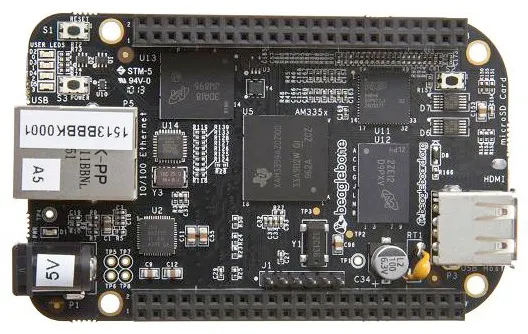BeagleBoard.org BeagleBone Black
×1USB Expansion HUB, USB 2.0 Powered
×1Test Accessory, USB Wi-Fi Dongle
×1

Software apps and online servicesOpenCV

Schematics

Our Car Setup (Front View)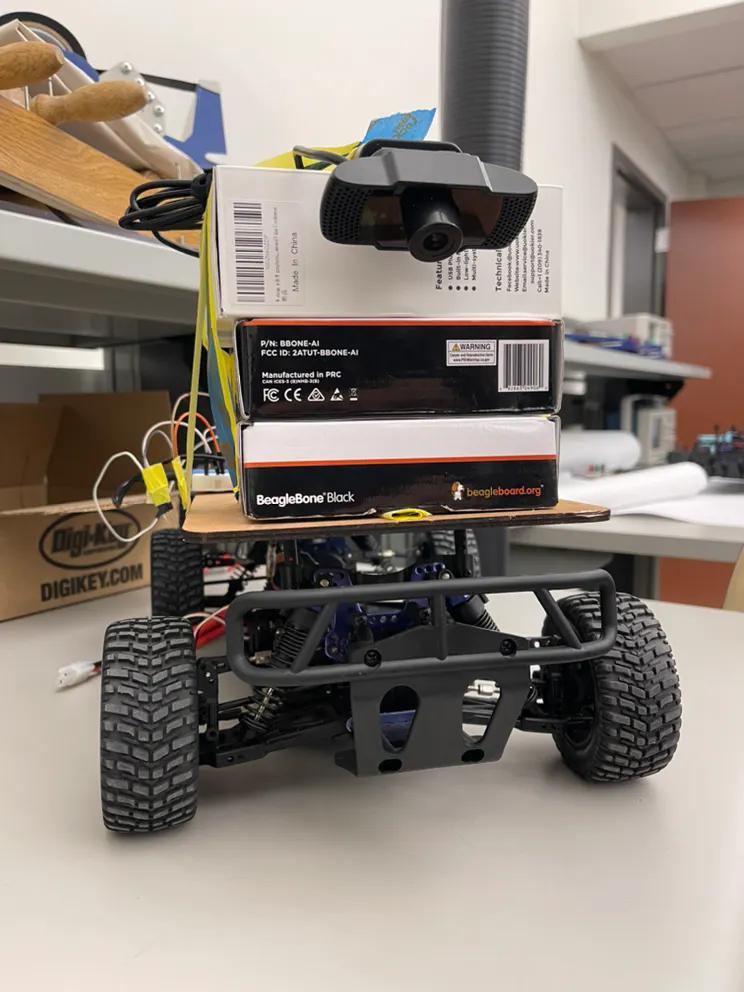Our Car Setup (Top View)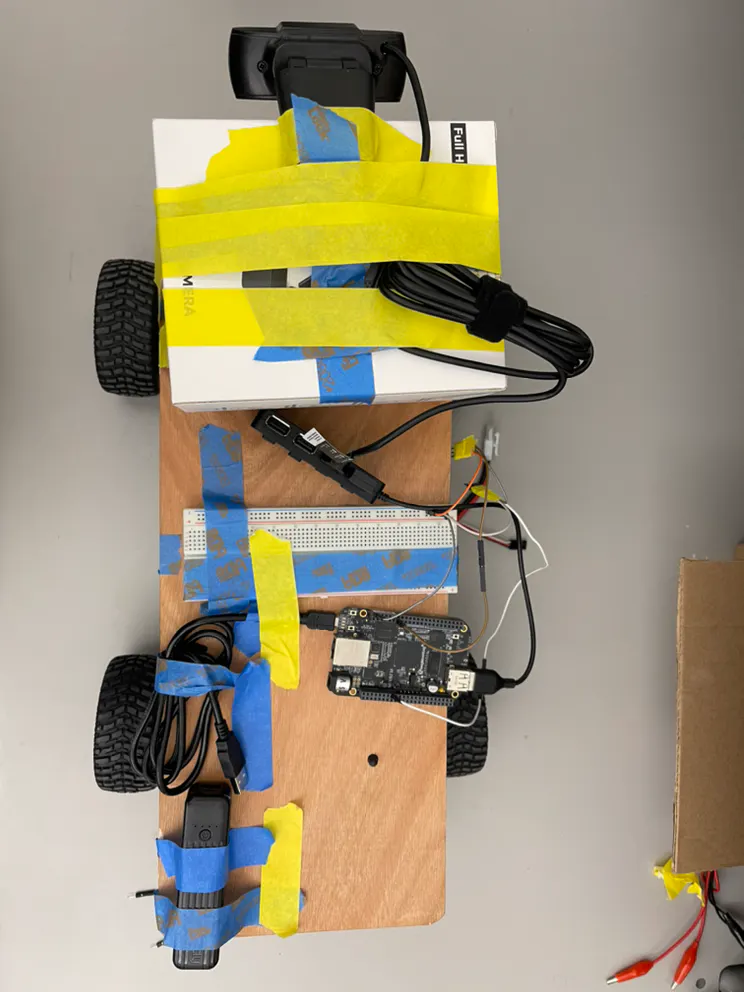Graph of Steering, Throttle and Error vs Frame Number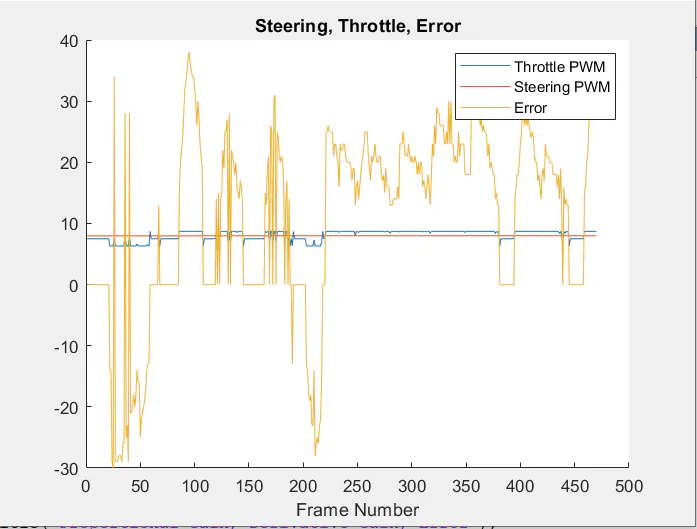Graph of Proportional Gain, Derivative Gain and Error vs Frame Number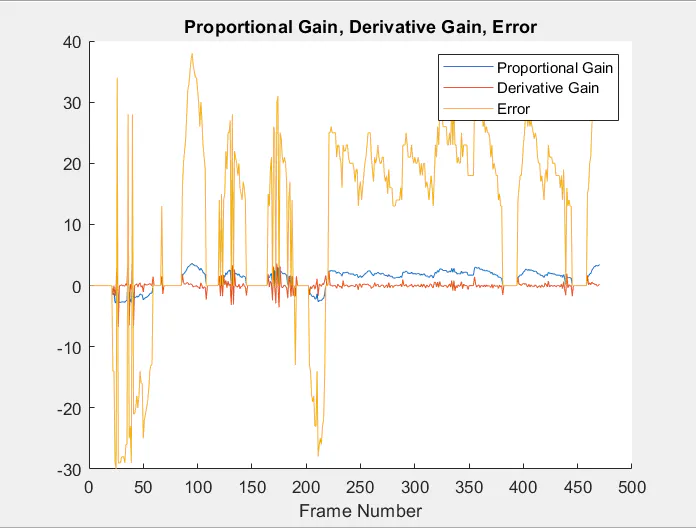Code

Code

Python
# Code based on user raja_961's instructable found here:
# https://www.instructables.com/Autonomous-Lane-Keeping-Car-Using-Raspberry-Pi-and/

import cv2
import numpy as np
import math
import sys
import time

# GPIO.setwarnings(False)

#throttle = physical pin 66
throttlePin = "P8_13" # Physical pin 22
# in3 = 23 # physical Pin 16
# in4 = 24 # physical Pin 18

#Steering
steeringPin = "P9_16" # Physical Pin 15
# in1 = 17 # Physical Pin 11
# in2 = 27 # Physical Pin 13

# Throttle
PWM.start(throttlePin,7.5, 50)
#PWM.set_duty_cycle(throttlePin,7.5)
# PWM.stop(throttlePin)

# Steering
PWM.start(steeringPin,7.5, 50)
#PWM.set_duty_cycle(steeringPin, 7.5)
# PWM.stop(steeringPin)

def detect_stop(hsv):
#takes image in hsv as input

#mask for the lower hue values
red_lower = np.array([0,50,50], dtype = "uint8")
red_higher = np.array([10,255,255], dtype = "uint8")
#find pixels that are within the mask range
#mask for the higher hue values
red_lower = np.array([170,50,50], dtype = "uint8")
red_higher = np.array([180,255,255], dtype = "uint8")
#find pixels within upper mask range

#get all pixels that are red
#sum up all values (0 if not red, 255 = logical 1 if red)

#if more than 3/8's of total screen (3/4s of bottom half) is red -> there is a "stop sign"
#return true if stop sign, false otherwise
return True if totalRed >= 80*60/9*1*255 else False

def detect_edges(hsv):
#changed to have hsv as input so that it doesnt need to be computed for edges and stops
# filter for blue lane lines
#cv2.imshow("HSV",hsv)
lower_blue = np.array([90, 120, 0], dtype = "uint8")
upper_blue = np.array([150, 255, 255], dtype="uint8")

# detect edges
#cv2.imshow("edges",edges)

return edges

def region_of_interest(frame):
#changed to find ROI from original frame
height, width, depth = frame.shape
cropped_frame = np.zeros_like(frame)
# only focus lower half of the screen
polygon = np.array([[
(0, height//2),
(width, height // 2),
(width, height - 1),
(0, height - 1)
]], np.int32)

# on image "mask", fill a square with corner points "polygon" with 1's
#blackens out top half of the screen
# cv2.imshow("roi",cropped_frame)

return cropped_frame

def detect_line_segments(cropped_edges):
rho = 1
theta = np.pi / 180
min_threshold = 10

line_segments = cv2.HoughLinesP(cropped_edges, rho, theta, min_threshold,
np.array([]), minLineLength=5, maxLineGap=150)

return line_segments

def average_slope_intercept(frame, line_segments):
lane_lines = []

if line_segments is None:
#print("no line segments detected")
return lane_lines

height, width,_ = frame.shape
left_fit = []
right_fit = []

boundary = 1/3
left_region_boundary = width * (1 - boundary)
right_region_boundary = width * boundary

for line_segment in line_segments:
for x1, y1, x2, y2 in line_segment:
if x1 == x2:
#print("skipping vertical lines (slope = infinity")
continue

fit = np.polyfit((x1, x2), (y1, y2), 1)
slope = (y2 - y1) / (x2 - x1)
intercept = y1 - (slope * x1)

if slope < 0:
if x1 < left_region_boundary and x2 < left_region_boundary:
left_fit.append((slope, intercept))
else:
if x1 > right_region_boundary and x2 > right_region_boundary:
right_fit.append((slope, intercept))

left_fit_average = np.average(left_fit, axis=0)
if len(left_fit) > 0:
lane_lines.append(make_points(frame, left_fit_average))

right_fit_average = np.average(right_fit, axis=0)
if len(right_fit) > 0:
lane_lines.append(make_points(frame, right_fit_average))

return lane_lines

def make_points(frame, line):
height, width, _ = frame.shape

slope, intercept = line

y1 = height  # bottom of the frame
y2 = int(y1 / 2)  # make points from middle of the frame down

if slope == 0:
slope = 0.1

x1 = int((y1 - intercept) / slope)
x2 = int((y2 - intercept) / slope)

return [[x1, y1, x2, y2]]

def display_lines(frame, lines, line_color=(0, 255, 0), line_width=6):
line_image = np.zeros_like(frame)

if lines is not None:
for line in lines:
for x1, y1, x2, y2 in line:
cv2.line(line_image, (x1, y1), (x2, y2), line_color, line_width)

line_image = cv2.addWeighted(frame, 0.8, line_image, 1, 1)

return line_image

def display_heading_line(frame, steering_angle, line_color=(0, 0, 255), line_width=5 ):
height, width, _ = frame.shape

steering_angle_radian = steering_angle / 180.0 * math.pi

x1 = int(width / 2)
y1 = height
x2 = int(x1 - height / 2 / math.tan(steering_angle_radian))
y2 = int(height / 2)

cv2.line(heading_image, (x1, y1), (x2, y2), line_color, line_width)

def get_steering_angle(frame, lane_lines):

height,width,_ = frame.shape

if len(lane_lines) == 2:
_, _, left_x2, _ = lane_lines
_, _, right_x2, _ = lane_lines
mid = int(width / 2)
x_offset = (left_x2 + right_x2) / 2 - mid
y_offset = int(height / 2)

elif len(lane_lines) == 1:
x1, _, x2, _ = lane_lines
x_offset = x2 - x1
y_offset = int(height / 2)

elif len(lane_lines) == 0:
x_offset = 0
y_offset = int(height / 2)

angle_to_mid_deg = int(angle_to_mid_radian * 180.0 / math.pi)
steering_angle = angle_to_mid_deg + 90

return steering_angle

video = cv2.VideoCapture(0)
video.set(cv2.CAP_PROP_FRAME_WIDTH,320)
video.set(cv2.CAP_PROP_FRAME_HEIGHT,240)

time.sleep(1)

##fourcc = cv2.VideoWriter_fourcc(*'XVID')
##out = cv2.VideoWriter('Original15.avi',fourcc,10,(320,240))
##out2 = cv2.VideoWriter('Direction15.avi',fourcc,10,(320,240))

speed = 7.96
lastTime = 0
lastError = 0

kp = 0.095
kd = kp * 0.07 #0.05
foundStop = False
red = 0
PWM.set_duty_cycle(throttlePin, speed)
PWM.set_duty_cycle(steeringPin, 7.5)

i = 0
while i < 300:
frame = cv2.resize(frame, (80, 60), interpolation=cv2.INTER_AREA)
# frame = cv2.flip(frame,-1)
if red > 1:
break
#cv2.imshow("original",frame)
#computed first so it doesnt have to be found each time for edges and stop sign detection
roi = region_of_interest(frame)
#moved outside detect edges to only convert roi frame once
hsv = cv2.cvtColor(roi, cv2.COLOR_BGR2HSV)

#check for stops
if detect_stop(hsv):
#makes sure it's detecting stop for the first time
#sleep for 3 seconds and set the speed to 0
PWM.set_duty_cycle(throttlePin, 7.5)
time.sleep(3)
#notes that we detected it for the first time, will not run again until another stop sign
foundStop = True
speed += .025
#print("STOOOOOOOOOOOOOOOOP")
red += 1
continue
else:
PWM.set_duty_cycle(throttlePin,speed)

#if no stop sign but foundStop is true -> we just passed a stop sign
elif foundStop:
#set foundStop to false for the next time
foundStop = False

PWM.set_duty_cycle(throttlePin,speed)
# print(i)
edges = detect_edges(hsv)
line_segments = detect_line_segments(edges)
lane_lines = average_slope_intercept(frame,line_segments)
lane_lines_image = display_lines(frame,lane_lines)
steering_angle = get_steering_angle(frame, lane_lines)

now = time.time()
dt = now - lastTime

deviation = steering_angle - 90
error = -1*deviation

if deviation < 13 and deviation > -13:
deviation = 0
error = 0
#    PWM.set_duty_cycle(steeringPin,7.5)

# elif deviation > 5:
#    PWM.set_duty_cycle(steeringPin,7.2)

# elif deviation < -5:
#     PWM.set_duty_cycle(steeringPin,7.9)

derivative = kd * (error - lastError) / dt
proportional = kp * (error)
PD = 7.5 + derivative + proportional
if PD > 8.7:
PD = 8.7
if PD < 6.3:
PD = 6.3

print(PD, speed, error, derivative, proportional)
if abs(error - lastError) <= 10 and i < 100: # 5
lastError = error
lastTime = time.time()
continue

PWM.set_duty_cycle(steeringPin,PD)
if abs(PD- 7.5) > .80:
PWM.set_duty_cycle(throttlePin,speed-.005)
else:
PWM.set_duty_cycle(throttlePin,speed)
lastError = error
lastTime = time.time()

#    out.write(frame)
i += 1
key = cv2.waitKey(1)
if key == 27:
break

PWM.set_duty_cycle(throttlePin,7.5)
PWM.set_duty_cycle(steeringPin,7.5)
#print("Reseted")

PWM.stop(throttlePin)
PWM.stop(steeringPin)
video.release()
##out.release()
##out2.release()
cv2.destroyAllWindows()
PWM.cleanup()

Credits

2 projects • 1 follower

Andrew Yan

2 projects • 1 follower

Angela Liu

2 projects • 1 follower

Katrina Ji

2 projects • 0 followers